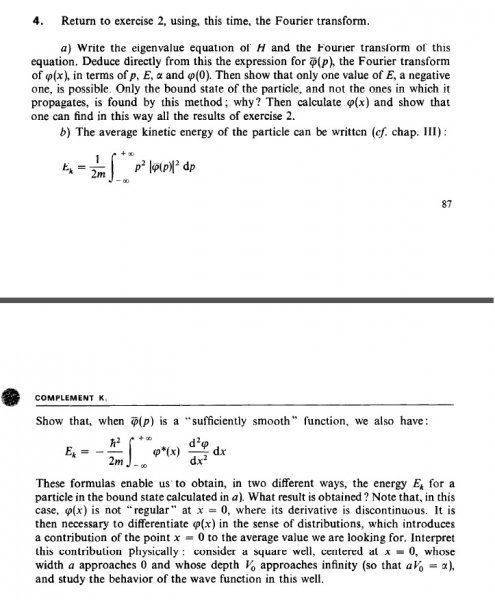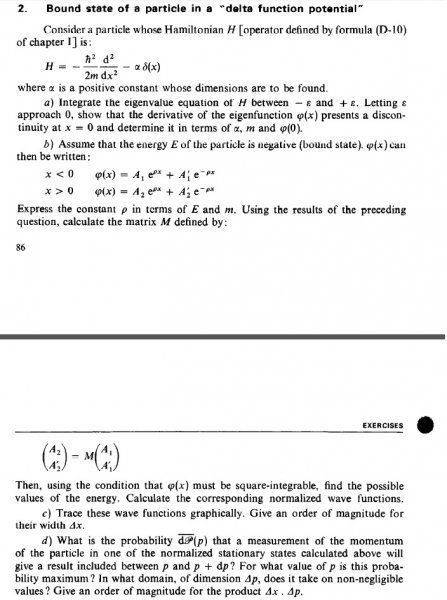# Cohen Tannoudji QM VOl.1 Chapter 1 Exercise 4 -- Eigenvalue & Fourier Transform of a Function

Helloaksdoq

## Homework Statement## Homework Equations## The Attempt at a Solution

I did Fourier transform directly to the eigenvalue equation and got

Psi(p)=a*Psi(0)/(p^2/2m-E)

But the rest, I don't even know where to start.
Any opinion guys?